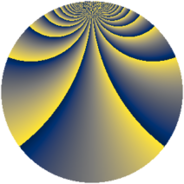# Properties

 Label 3630.2.bgLevel $3630$ Weight $2$ Character orbit 3630.bg Rep. character $\chi_{3630}(43,\cdot)$ Character field $\Q(\zeta_{44})$ Dimension $2640$ Sturm bound $1584$

# Related objects

## Defining parameters

 Level: $$N$$ $$=$$ $$3630 = 2 \cdot 3 \cdot 5 \cdot 11^{2}$$ Weight: $$k$$ $$=$$ $$2$$ Character orbit: $$[\chi]$$ $$=$$ 3630.bg (of order $$44$$ and degree $$20$$) Character conductor: $$\operatorname{cond}(\chi)$$ $$=$$ $$605$$ Character field: $$\Q(\zeta_{44})$$ Sturm bound: $$1584$$

## Dimensions

The following table gives the dimensions of various subspaces of $$M_{2}(3630, [\chi])$$.

Total New Old
Modular forms 16000 2640 13360
Cusp forms 15680 2640 13040
Eisenstein series 320 0 320

## Trace form

 $$2640q + 16q^{5} + O(q^{10})$$ $$2640q + 16q^{5} - 44q^{10} - 8q^{11} - 88q^{13} - 8q^{15} + 264q^{16} - 84q^{22} - 16q^{23} - 16q^{26} - 16q^{31} + 12q^{33} + 264q^{36} + 232q^{37} + 8q^{42} + 32q^{47} + 48q^{53} + 52q^{55} + 16q^{56} + 8q^{58} + 16q^{60} + 8q^{66} - 336q^{67} - 112q^{70} - 32q^{75} + 88q^{76} - 16q^{80} - 2640q^{81} - 32q^{82} - 352q^{85} - 32q^{86} + 4q^{88} - 72q^{92} - 16q^{93} + 24q^{97} + O(q^{100})$$

## Decomposition of $$S_{2}^{\mathrm{new}}(3630, [\chi])$$ into newform subspaces

The newforms in this space have not yet been added to the LMFDB.

## Decomposition of $$S_{2}^{\mathrm{old}}(3630, [\chi])$$ into lower level spaces

$$S_{2}^{\mathrm{old}}(3630, [\chi]) \cong$$ $$S_{2}^{\mathrm{new}}(605, [\chi])$$$$^{\oplus 4}$$$$\oplus$$$$S_{2}^{\mathrm{new}}(1210, [\chi])$$$$^{\oplus 2}$$$$\oplus$$$$S_{2}^{\mathrm{new}}(1815, [\chi])$$$$^{\oplus 2}$$